### Home > CALC > Chapter 7 > Lesson 7.3.3 > Problem7-135

7-135.
1.Evaluate each of the following integrals. Homework Help ✎

1.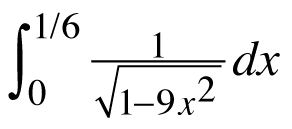2.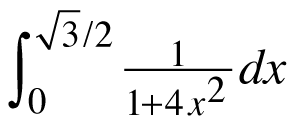3.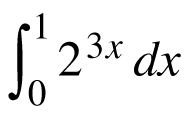4.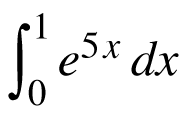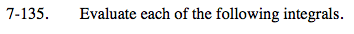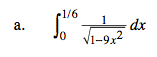$\int_{0}^{\frac{1}{6}}\frac{1}{\sqrt{1-(3x)^{2}}}dx$

What trig derivative do you recognize?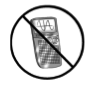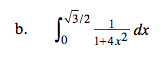Refer to the hint in part (a).
Also, remember that 4x² = (2x

$=\frac{1}{3\ln 2}2^{3x}\Big|_0^1=\frac{7}{3\ln 2}$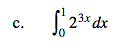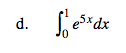This integral is very much like that in part (c).